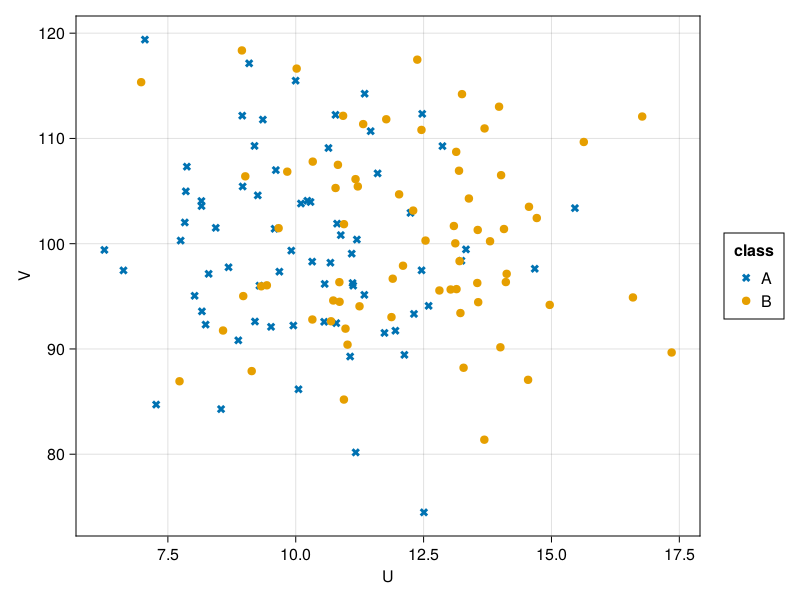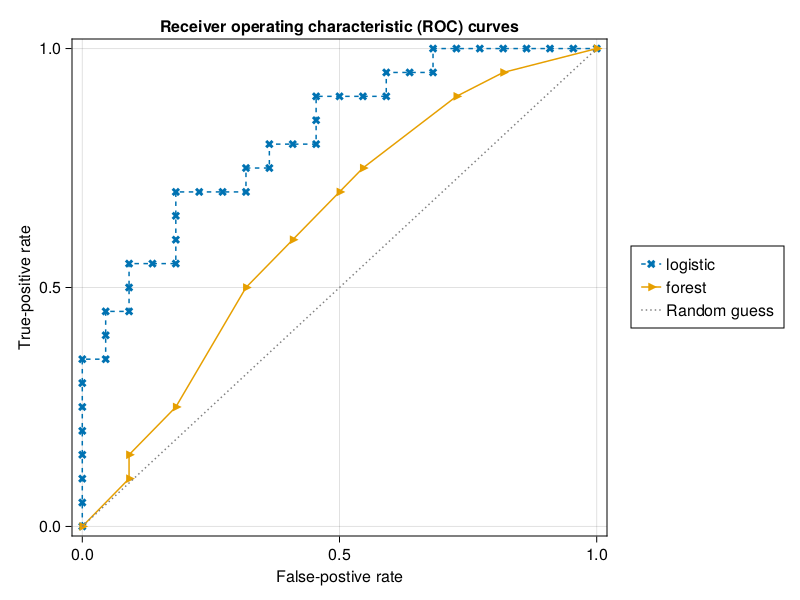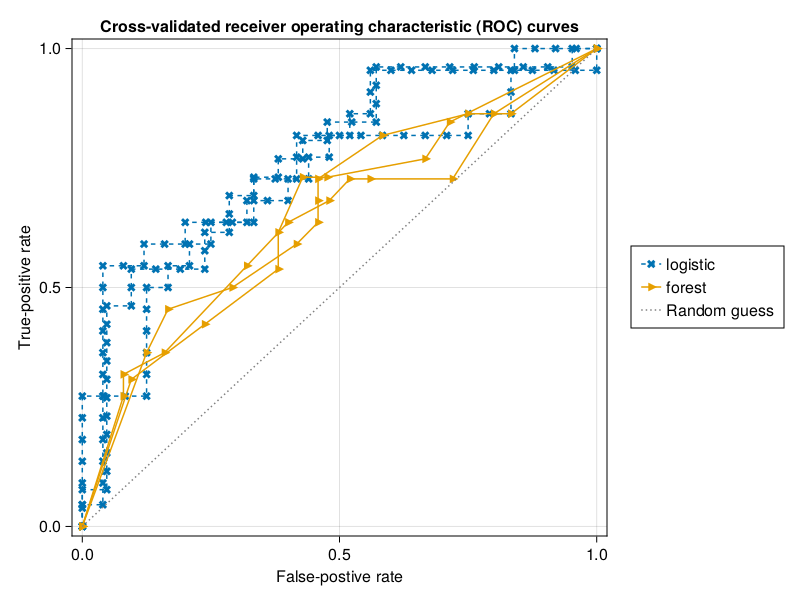HUIJZER.XYZ

# Random forest classification in Julia

2021-01-21

Below is example code for fitting and evaluating a linear regression and random forest classifier in Julia. I've added the linear regression as a baseline for the random forest. The models are evaluated on a mock variable $U$ generated from two distributions, namely

\begin{aligned} d_1 &= \text{Normal}(10, 2) \: \: \text{and} \\ d_2 &= \text{Normal}(12, 2), \end{aligned}

The random variable $V$ is just noise meant to test the classifier, generated via

$$V \sim \text{Normal}(100, 10)$$

This data isn't meant to show that random forests are good classifiers per se. It is just meant to show how to fit and plot random forests in Julia. One way to show that random forests are accurate would be to have about the same or more variables than observations (Biau & Scornet, 2016).

## Data generation

Let's load some packages and generate the data:

begin
import MLJGLMInterface
import MLJDecisionTreeInterface

using CairoMakie
using CategoricalArrays
using Colors: RGB
using DataFrames
using Distributions
using MLJBase
using MLJ
using StableRNGs: StableRNG
using Random
end
classlabels = ["A", "B"];
df = let
# Number of elements per class.
n = 70
μ1 = 10
μ2 = 12
σ = 2

d1 = Normal(μ1, σ)
d2 = Normal(μ2, σ)

Random.seed!(12)
classes = repeat(classlabels, n)

df = DataFrame(
class = categorical(classes),
U = [class == "A" ? rand(d1) : rand(d2) for class in classes],
V = rand(Normal(100, 10), 2n)
)
end
classUV
1"A"10.677798.1947
2"B"14.0715101.393
3"A"7.0505119.386
4"B"17.349789.6641
5"A"8.2968997.1358
6"B"11.246994.0527
7"A"9.9129799.3432
8"B"15.6322109.662
9"A"8.9616105.435
10"B"13.6919110.949
...
140"B"13.2293.4114
X = (; df.U, df.V);
y = df.class;

If we plot this, we can see that the points of "B" lie more to the right. More specifically, the points for "B" are higher on average for U:

let
fig = Figure()
ax = Axis(fig[1, 1]; xlabel="U", ylabel="V")
classmarkers = [:xcross, :circle]
for (label, marker) in zip(classlabels, classmarkers)
filtered = filter(:class => ==(label), df)
scatter!(ax, filtered.U, filtered.V; label, marker)
end
Legend(fig[1, 2], ax, "class")
fig
end## Train and test split

Let's split our data before continuing. Training and evaluating (testing) on the same data is not great because we want to know how well our model generalizes. It is easy to make correct predictions when you have seen the data which you need to predict already. For more information, see topics such as overfitting. So, to avoid this problem, we split the data up in a train and test set.

train, test = let
rng = StableRNG(123)
MLJ.partition(eachindex(df.class), 0.7; shuffle=true, rng)
end;

## Model fitting

Now, we can fit a model in order to determine the accuracy later:

logistic = let
LinearBinary = @load LinearBinaryClassifier pkg=GLM verbosity=0
LinearBinary()
end;
fitted_logistic = let
Random.seed!(11)
mach = machine(logistic, X, y)
fit!(mach; rows=train)
mach
end;
r2(x) = round(x; digits=2);
coefficients = r2.(fitted_params(fitted_logistic).coef)
2-element Vector{Float64}:
0.44
0.03

The second coefficient in the linear model is close to zero. This is exactly what the model should do since V is random noise.

Let's also fit the random forest model:

forest = let
Random.seed!(11)
DecisionTree = @load DecisionTreeClassifier pkg=DecisionTree verbosity=0
tree = DecisionTree()
EnsembleModel(tree; n=10)
end;

## Accuracy

Now that we know how to fit the models and verified the linear model, we can compare the accuracies and plot the receiver operating characteristic (ROC) curves. In this curve, higher means a better predictive performance.

Here, I've used Makie.jl instead of AlgebraOfGraphics.jl. This is more barebones, that is, I had to write code to smooth the line. This took a bit of extra time upfront, but allows for much greater flexibility which can save time in the long run.

The next function fits a model and obtains the false-positive rates fprs and the true-postive rates tprs:

function fprs_tprs(model, X, y, train, test)
mach = machine(model, X, y)
fit!(mach; rows=train)
predictions = MLJ.predict(mach; rows=test)
fprs, tprs, _ = roc_curve(predictions, df.class[test])
return fprs, tprs
end;

For plotting, I'm first defining a struct here. Objects of this RocCurve type can be sent to the plotting function in order to show multiple curves in one plot. I'm using a struct like this because it makes things more explicit.

You can ignore the details of the code here and feel free to copy and adjust the code for your usage.

begin
struct RocCurve
fprs::Vector{Float64}
tprs::Vector{Float64}
label::String
linestyle::Union{Nothing,Symbol}
marker::Symbol
color::RGB
end

function RocCurve(model::MLJ.Model, label::String, linestyle, marker, color)
# Using the train-test split defined above.
fprs, tprs = fprs_tprs(model, X, y, train, test)
return RocCurve(fprs, tprs, label, linestyle, marker, color)
end
end;
curves = [
RocCurve(logistic, "logistic", :dash, :x, wongcolors),
RocCurve(forest, "forest", nothing, :rtriangle, wongcolors)
];
plot_curves(curves)From this plot, we can learn that the logistic model has a higher area under the curve meaning that it is a better predictive model on our single train test split. This makes sense because the random forest is very likely to overfit our data, that it, it will fit patterns which hold for the test set but do not necessarily hold in general.

However, it could be that the conclusion would be different if the train test split was slightly different. To draw more robust conclusions, we need cross-validation.

## K-fold cross-validation

By doing a train and test split, we basically threw a part of the data away. For small datasets, like the dataset in this example, that is not very efficient. Therefore, we also do a k-fold cross-validation. This has the benefit that we use all the data that we have for evaluations. We can evaluate the model via the evaluate function from MLJ.jl:

function evaluate_model(model)
rng = StableRNG(8)
resampling = CV(; nfolds=10, shuffle=true, rng)
evaluations = evaluate(model, X, y; resampling, measure=auc, verbosity=0)
average = r2(only(evaluations.measurement))
per_fold = r2.(only(evaluations.per_fold))
return (; average, per_fold)
end;
evaluate_model(logistic)
(average = 0.75,
per_fold = [0.69, 0.83, 0.92, 0.88, 0.61, 0.56, 0.88, 0.87, 0.88, 0.42],)
evaluate_model(forest)
(average = 0.62,
per_fold = [0.65, 0.77, 0.81, 0.72, 0.59, 0.67, 0.8, 0.53, 0.38, 0.28],)

So, our previous conclusion still holds because the average is higher for the logistic model. Still, it would be nice to see plots instead of numbers. Let's make them.

## ROC curves for k-fold cross-validation

In scikit-learn, there is a function to create roc plots with cross-validation. Well, there is good news and bad news. The bad news is, scikit-learn is a Python library and making Julia and Python work together is difficult to set up if you want to run your code locally and in CI jobs. The good news is, thanks to Pluto.jl, MLJ.jl and Makie.jl, it shouldn't be too hard to make such a plot ourselves in pure Julia.

The MLJ.jl API only returns the AUC scores per fold and not the number of true and false-positives. We need those to be able to plot the ROC curves. To work around this, we can pull the train-test rows from evaluations. Next, we have to fit the models on those train-test rows, get the true and false-positives and plot them:

function cross_validated_fprs_tprs(model)
rng = StableRNG(7)
resampling = CV(; nfolds=3, shuffle=true, rng)
Random.seed!(12)
evaluations = evaluate(model, X, y; resampling, measure=auc, verbosity=0)
return map(evaluations.train_test_rows) do (train, test)
fprs_tprs(model, X, y, train, test)
end
end;

To combine the results of the multiple cross-validation runs, I've put all the false and true-positive rates in one big vector:

It would be nicer to use the mean of each of the n runs in each cross-validation 240split. Unfortunately that was impossible for the random forest for which n was not constant over the splits. I suspect that the difference is caused by the forest model being unable to fit on some splits.

We can convert these combined fprs and tprs to RocCurves and plot them:

function cross_validation_curve(model, label::String, linestyle, marker, color)
FPRS_TPRS = cross_validated_fprs_tprs(model)
subset = FPRS_TPRS[1:3]
return map(subset) do fprs_tprs
fprs = first(fprs_tprs)
tprs = last(fprs_tprs)
return RocCurve(fprs, tprs, label, linestyle, marker, color)
end
end;
cv_curves = [
cross_validation_curve(logistic, "logistic", :dash, :x, wongcolors);
cross_validation_curve(forest, "forest", nothing, :rtriangle, wongcolors)
];
let
title = "Cross-validated receiver operating characteristic (ROC) curves"
plot_curves(cv_curves; title, show_points=true)
endNoice! There are large differences between the models which makes sense. This is the nice thing about cross-validation. It can give an idea of the unstability of the models. In other words, it's easy to take a single fit as a source of truth while in fact things are much more uncertain. If you want to see more stable outcomes, download this notebook and increase n to get more data.

## Appendix

# Thanks to AlgebraOfGraphics.jl
wongcolors = [
RGB(0/255, 114/255, 178/255), # blue
RGB(230/255, 159/255, 0/255), # orange
RGB(0/255, 158/255, 115/255), # green
RGB(204/255, 121/255, 167/255), # reddish purple
RGB(86/255, 180/255, 233/255), # sky blue
RGB(213/255, 94/255, 0/255), # vermillion
RGB(240/255, 228/255, 66/255), # yellow
];
function plot_curves(
curves::Vector{RocCurve};
xlabel="False-postive rate",
ylabel="True-positive rate",
show_points::Bool=true
)
fig = Figure()
ax = Axis(fig[1, 1]; title, xlabel, ylabel)

plotted = []
for curve in curves
fprs = curve.fprs
tprs = curve.tprs
line = lines!(ax, fprs, tprs; curve.linestyle, curve.color)
objects = []
if show_points
scat = scatter!(ax, fprs, tprs; markersize=12, curve.marker, curve.color)
objects = [scat, line]
else
objects = [line]
end
if !(curve.linestyle in getproperty.(plotted, :linestyle))
nt = (; curve.linestyle, objects, curve.label)
push!(plotted, nt)
end
end
random = lines!(ax, 0:1, 0:1; color=:gray, linestyle=:dot)
push!(plotted, (; linestyle=:dot, objects=[random], label="Random guess"))
ylims!(ax, -0.02, 1.02)
xlims!(ax, -0.02, 1.02)

Legend(
fig[1, 2],
getproperty.(plotted, :objects),
getproperty.(plotted, :label)
)
return fig
end;

Built with Julia 1.9.4 and

CairoMakie 0.10.5
CategoricalArrays 0.10.8
Colors 0.12.10
DataFrames 1.5.0
Distributions 0.25.93
MLJ 0.19.1
MLJBase 0.21.11
MLJDecisionTreeInterface 0.4.0
MLJGLMInterface 0.3.5
StableRNGs 1.0.0

To run this blog post locally, open this notebook with Pluto.jl.